# Ionic Equilibrium Formulas for NEET

Most of the chemical reactions occur in solutions when a given substance is dissolved in the solvents like water. In an ionic solution the substance splits up into ions whereas in molecular solution, the substance remains as such. Substance can be classified into the following types, nonelectrolytes and electrolytes. Electrolytes conduct electricity in aqueous solution or molten state. Non-electrolyte do not conduct electricity in aqueous solution or molten state.

## Ostwald Dilution Law

Ostwald pointed out that the law of chemical equilibrium is applicable to ionic equilibrium.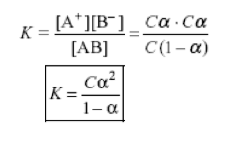## pH Scale

pH scale was introduced by Sorenson to measure acidity or basicity of a solution. pH stands for potential or concentration of H+ ion.

pH of strong acid or base does not depend upon temperature. pH of weak acid decreases with increase in temperature due to increase in ionization. pH of weak base increases with increase in temperature due to increase in ionization or [OH-] ion concentration.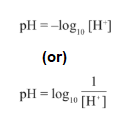## pOH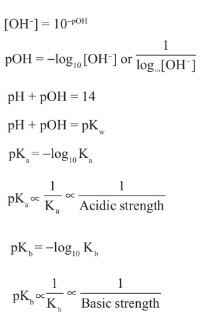## Properties of Water

In pure water

H+ ions = OH ions, so it is neutral.

Molar concentration / Molarity of water = 55.56M

## Ionic Product of Water

Ionic product of water is the product of the molar concentrations of H+ or H3O+ and OH- ions. It is denoted by Kw.

Kw = [H3O+].[OH]

Kw = ionic product of water

Kw – Ka x Kb

pKw = pKa + pKb

At 25oC,

Kw = 1 x 10-14

pKw = 14

The value of Kw increases with increase in temperature.

## Degree of Dissociation of Water

Degree of ionization is the extent to which an electrolyte gets ionized in a solvent. It is denoted by ɑ.

ɑ = number of molecules dissociated/Total number of molecules.

The degree of dissociation of weak electrolyte is directly proportional to dilution that is alpha maximum at infinite dilution.

## Buffer Solution

Buffer solution is the solution whose pH in addition to a small amount of strong acid or base does not change much. It is also called reserve acidity or basicity of the solution, as it resists change in pH value and this action to resist pH change is called buffer action.

Buffer Capacity is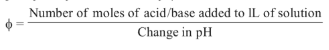## Solubility

Solubility is the weight of solute in grams present in 100g of solvent. It is denoted by s and is expressed in mole per litre or gram per litre.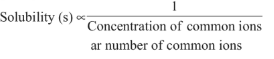## Solubility Product

The solubility product constant Ksp is the equilibrium constant for the equilibrium established between a slightly soluble ionic compound and its ions in a saturated aqueous solution.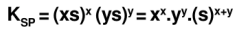## Salt Hydrolysis

When salt is added to water ions of the salt interact with water to cause acidity or basicity in aqueous solution. This ionic interaction is called salt hydrolysis.

### Degree of Hydrolysis

h = Number of moles of salt hydrolyzed/Total moles of the salt taken.

### Salt of weak acid & strong base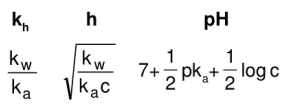### Salt of strong acid & weak base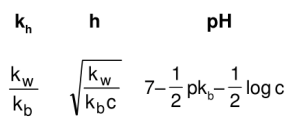### Salt of weak acid & weak base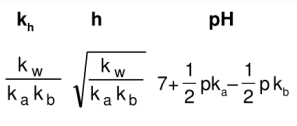### Salt of strong acid & strong base

Do not hydrolyse

pH = 7

Ionic equilibrium is the study of weak electrolyte and its effect on weak electrolyte. Weak electrolyte dissociates negligibly because balance exists between its ion and electrolyte so the study of weak electrolytes is generally known as ionic equilibrium and the effect of strong electrolyte on weak electrolyte.

1. Lok Jeevan

Thank you so much Byju’s

2. Rahul Kumar Singh

Fantastic

3. Katyayini

Nice notes

4. Thank u for the formulae Byjus